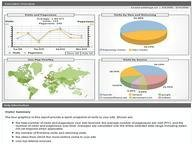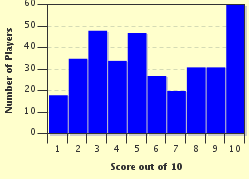FREE! Click here to Join FunTrivia. Thousands of games, quizzes, and lots more!# Dice Probabilities Trivia Quiz

### All questions will deal with dice rolls. Be careful - some involve conditional probability! Good Luck!

A multiple-choice quiz by rodney_indy. Estimated time: 4 mins.

Author
rodney_indy
Time
4 mins
Type
Multiple Choice
Quiz #
268,305
Updated
Dec 03 21
# Qns
10
Difficulty
Tough
Avg Score
5 / 10
Plays
1596
Last 3 plays: Guest 136 (0/10), Guest 163 (2/10), Guest 175 (2/10).
This quiz has 2 formats: you can play it as a or as shown below.
Scroll down to the bottom for the answer key.
1. Two six-sided dice are rolled. Find the probability the first die came up a 2 and the second die came up a 5. Hint

1/36
1/12
1/18
1/9

#### NEXT>

2. Two six-sided dice are rolled. Find the probability that one die came up a 2 and the other came up a 6. Hint

1/36
1/18
1/12
1/9

#### NEXT>

3. Two six-sided dice are rolled. Find the probability that at least one die is a 3. Hint

1/3
1/2
11/36
1/6

#### NEXT>

4. Two six-sided dice are rolled. Find the probability that the sum is 8. Hint

1/3
5/36
3/16
5/18

#### NEXT>

5. Two six-sided dice are rolled. Find the probability that the sum is 8 given that the first die was 3. Hint

1/12
1/5
1/6
5/18

#### NEXT>

6. Two six-sided dice are rolled. Find the probability that the first die came up 3 given that the dice sum to 8. Hint

5/18
1/6
1/5
1/12

#### NEXT>

7. Two six-sided dice are rolled. Find the probability that their sum is not 7. Hint

3/4
5/6
2/3
23/36

#### NEXT>

8. Two six-sided dice are rolled. Find the probability that their sum is not 8, given that their sum is not 7. Hint

5/6
2/3
3/4
23/36

#### NEXT>

9. Two six-sided dice are rolled. Find the probability that the first die is not a 5, given that the second die is not a 2. Hint

2/3
5/6
25/36
29/36

#### NEXT>

10. Two six-sided dice are rolled. But this time, the dice aren't fair: For each die, a 1 is twice as likely to be rolled as a 2, a 2 is twice as likely to be rolled as a 3, ..., and a 5 is twice as likely to be rolled as a 6 (in other words, each number is twice as likely as the number that follows it). So what is the probability of rolling a sum of 7? Hint

64/1223
64/1023
64/1123
64/1323

 (Optional) Create a Free FunTrivia ID to save the points you are about to earn:Select a User ID:Choose a Password:Your Email:

Most Recent Scores
Sep 13 2023 : Guest 136: 0/10
Sep 11 2023 : Guest 163: 2/10
Sep 07 2023 : Guest 175: 2/10
Sep 06 2023 : Guest 199: 3/10
Sep 05 2023 : Guest 1: 3/10
Sep 04 2023 : Guest 102: 7/10
Sep 02 2023 : Guest 68: 6/10
Aug 21 2023 : Guest 97: 9/10
Aug 20 2023 : Guest 110: 4/10

Score DistributionQuiz Answer Key and Fun Facts
1. Two six-sided dice are rolled. Find the probability the first die came up a 2 and the second die came up a 5.

There are 6 possibilities for the first die and 6 possibilities for the second die. Hence there are 6 * 6 = 36 possible dice rolls. There is only one of them: (2,5) which has 2 on the first die and 5 on the second die. Thus the probability is 1/36.
2. Two six-sided dice are rolled. Find the probability that one die came up a 2 and the other came up a 6.

This event consists of the outcomes (2,6), (6,2). Hence it has probability 2/36 = 1/18.
3. Two six-sided dice are rolled. Find the probability that at least one die is a 3.

The probability that the first die is 3 is 1/6, the probability that the second die is 3 is 1/6, and the probability that both dice are 3 is 1/36. By the principle of inclusion/exclusion, the probability that the first die or the second die is 3 is just 1/6 + 1/6 - 1/36 = 11/36. This probability is useful in backgammon - this is the probability of entering off the bar when there is only one open point in the opponent's table.
4. Two six-sided dice are rolled. Find the probability that the sum is 8.

The outcomes in the event "the sum is 8" are: (2,6), (3,5), (4,4), (5,3), and (6,2). Since there are 5 ways the sum can be 8, the probability is 5/36.
5. Two six-sided dice are rolled. Find the probability that the sum is 8 given that the first die was 3.

This is a conditional probability question. We are given that the first die was 3: (3,1), (3,2), (3,3), (3,4), (3,5), (3,6)

Of these 6 possibilities, (3,5) is the only outcome where the dice sum to 8. So the probability is 1/6.
6. Two six-sided dice are rolled. Find the probability that the first die came up 3 given that the dice sum to 8.

This is another conditional probability question. We are given that the dice sum to 8: (2,6), (3,5), (4,4), (5,3), (6,2)
Of the 5 ways that the dice sum to 8, only one has the first die 3: (3,5). Hence the probability is 1/5.
7. Two six-sided dice are rolled. Find the probability that their sum is not 7.

The ways in which a 7 can be rolled are: (1,6), (2,5), (3,4), (4,3), (5,2), and (6,1). There are 6 ways a sum of 7 can be rolled. There are 36 total dice rolls, thus there are 30 ways a sum of 7 cannot be rolled. Hence the probability is 30/36 = 5/6.
8. Two six-sided dice are rolled. Find the probability that their sum is not 8, given that their sum is not 7.

We are given that the sum is not 7. In question number 7, we saw that there were 30 ways the sum could not be 7. The ways we can roll a sum of 8 are: (2,6), (3,5), (4,4), (5,3), and (6,2). So among the 30 ways we cannot roll a sum of 7, there are 25 ways we cannot roll a sum of 8. Hence the probability is 25/30 = 5/6.
9. Two six-sided dice are rolled. Find the probability that the first die is not a 5, given that the second die is not a 2.

Let E be the event "the first die is a 5" and F be the event "the second die is a 2." E and F are independent events. Hence so are the complements of E and F. This means that the probability of the complement of E given the complement of F is just the probability of the complement of E, which is 1 - 1/6 = 5/6.
10. Two six-sided dice are rolled. But this time, the dice aren't fair: For each die, a 1 is twice as likely to be rolled as a 2, a 2 is twice as likely to be rolled as a 3, ..., and a 5 is twice as likely to be rolled as a 6 (in other words, each number is twice as likely as the number that follows it). So what is the probability of rolling a sum of 7?

Let x = the probability of rolling a 6. Then 2x is the probability of rolling a 5, 2*2x = 4x = the probability of rolling a 4, 8x = the probability of rolling a 3, 16x = the probability of rolling a 2, and 32x = the probability of rolling a 1. These are all the outcomes, so these probabilities must sum to 1: x + 2x + 4x + 8x + 16x + 32x = 1 which simplifies to 63x = 1 which means x = 1/63. So Pr(1) = 32/63, Pr(2) = 16/63, Pr(3) = 8/63, Pr(4) = 4/63, Pr(5) = 2/63, and Pr(6) = 1/63. There are six ways to roll a sum of 7:

(1,6), (2,5), (3,4), (4,3), (5,2), (6,1)

By independence, their corresponding probabilities are

(32/63)*(1/63), (16/63)*(2/63), (8/63)*(4/63), (4/63)*(8/63), (2/63)*(16/63), and (1/63)*(32/63).

Each probability is 32/63^2. We add up these six probabilities to get the probability of rolling a sum of 7: 6*(32/63^2) = 64/1323.

I hope you enjoyed my quiz! Thanks for playing!
Source: Author rodney_indy

This quiz was reviewed by FunTrivia editor crisw before going online.
Any errors found in FunTrivia content are routinely corrected through our feedback system.